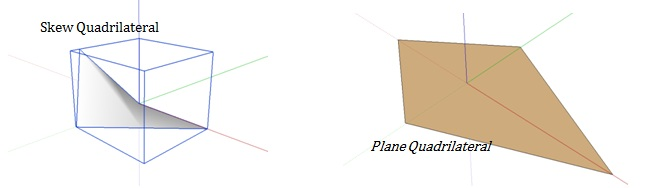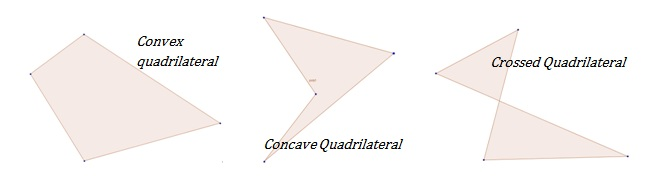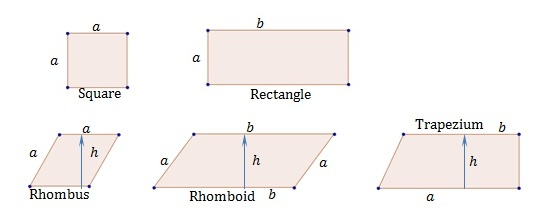# How to Find the Area of Quadrilaterals

Knowing how to find the area of quadrilaterals, is a fundamental knowledge required in mathematical measurements. Quadrilateral is a polygon with four sides. It is sometimes called as quadrangle or tetragon. Usually the four vertices are considered to lie on the same plane. However, when they are not lying on the same plane, it is known as a skew quadrilateral.Quadrilaterals are divided into three categories based on the position of vertices and the sides. If all the external angles of a quadrilateral are reflex angles, it is called a convex quadrilateral. If any of the external angles of a quadrilateral is not reflex angles, that quadrilateral is a concave quadrilateral. If the sides of the quadrilateral intersect at appoint, it is known as a crossed quadrilateral.Some quadrilaterals with regular shapes are listed below.Area of each shape can be found using formulas in the following section.

Square, rectangle, rhombus and the rhomboid all are parallelograms. Therefore, their opposing sides are parallel and equal. Square has all equal sides and all internal angles as right angles, and the rectangle has unequal adjacent sides, but all internal angles are right angles. Rhombus has equal sides with oblique, internal angles. In the case of the rhomboid not only the adjacent sides are different and internal angles are oblique.

Trapezium is not a parallelogram, and only two of the sides are parallel. Parallel sides are unequal in length and the separation between the parallel sides is considered as the height of the trapezium.

## Find the Area of the Quadrilaterals – Area formulas

For finding the area of the square only the length of a side is required, and for the rectangle, lengths of both sides are required.

### Area of Square – Formula

Area of a Square = a2  where a is the length of the sides

### Area of a Rectangle – Formula

Area of a Rectangle = a × b where a and b are the lengths of the rectangles

### Area of a Rhombus – Formula

For both rhombus and rhomboid, the length of a side and the perpendicular height from that side are required.

Area of a Rhombus = a × h where a and h are the side length and height of the rhombus respectively

Area of a Rhomboid = a × h where a and h are the side length and height of the rhomboid respectively

### Area of a Trapezium – Formula

For trapezium, length of both parallel sides and the perpendicular height is needed.

Area of a Trapezium = ½ (a + b) × where a and b are the length of both parallel sides and h is the perpendicular height

## Find the Area of Quadrilaterals – Examples

• The side of a square is 10cm. Find the area of the square.

Using the square are formula,

A Square a= 10= 100cm2

• A piece of land has a length of 700m and a width of 120m, what is the total area of the land?

Using the rectangle area formula,

A Rectangle = a×= 700×120 = 84000m2

• A rhombus has sides with length 5cm and two adjacent sides make an angle of 30 degrees, what is the area of the rhombus?

Using the rhombus area formula,

ARhombus = a×h = 5×5sin 300 = 12.5m2

• A rhomboid has sides with length of sides are twice the width. If the perimeter of the figure is 24cm and it makes a pair of 1200 internal angles, find the area of the rhomboid.

Length of the sides are not given, but a relation between the length and the width is given and the perimeter. Therefore, we can deduce the sides’ length by that.

If width is x, then the length is 2x. Then, the perimeter is + 2+ + 2= 24, and the solution gives x=4cm.

Since the rhomboid makes an angle 1200 at a vertex, the area is,

Using the rhomboid area formula,

Arhomboid = a×h = 4×4sin (1800-1200 ) = 4×4×√3/2〗= 8√3 = 8×1.73 = 13.85cm2